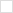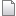TWiki>VerilogAMS Web>AmsExamples>AmsExamplesDCM (2012-09-14, DavidMiller)

# Digital Component Models (DCM)

Type Description Source
Logic AND 'and' module with variable number of input terminals and.va
Logic NAND 'nand' module with variable number of input terminals nand.va
Logic OR 'or' module with variable number of input terminals or.va
Logic NOR 'nor' module with variable number of input terminals nor.va
Logic XOR 'xor' module with variable number of input terminals xor.va
Logic XNOR 'xnor' module with variable number of input terminals xnor.va
Logic NOT Inverter module with variable number of input and output terminals not.va
D-Flip Flop D-Flip Flop d_ff.va
JK-Flip Flop JK-Flip Flop jk_ff.va
RS-Flip Flop RS-Flip Flop rs_ff.va
T-Flip Flop T-Flip Flop t_ff.va
Clock Generator Clock generator for symmetric clock clock_generator.va
Bit Error Rate Bit error rate calculator model biterrorrate.va

## Logic AND

 Module Name V_and in: terminal : input [0:size-1] voltage out: terminal : output voltage size: number of input bits = 2 from [2:inf) vout_high: high output voltage = 5.0 vout_low: low output voltage = 0.0 from (-inf:vout_high) vth: input threshold voltage = 1.4 tdelay: time between change on a input and output : 5n from [0:inf) trise: rising time = 1n from [0:inf) tfall: falling time = 1n from [0:inf)

## Logic NAND

 Module Name V_nand in: terminal : input [0:size-1] voltage out: terminal : output voltage size: number of input bits = 2 from [2:inf) vout_high: high output voltage = 5.0 vout_low: low output voltage = 0.0 from (-inf:vout_high) vth: input threshold voltage = 1.4 tdelay: time between change on a input and output : 5n from [0:inf) trise: rising time = 1n from [0:inf) tfall: falling time = 1n from [0:inf)

## Logic OR

 Module Name V_or in: terminal : input [0:size-1] voltage out: terminal : output voltage size: number of input bits = 2 from [2:inf) vout_high: high output voltage = 5.0 vout_low: low output voltage = 0.0 from (-inf:vout_high) vth: input threshold voltage = 1.4 tdelay: time between change on a input and output : 5n from [0:inf) trise: rising time = 1n from [0:inf) tfall: falling time = 1n from [0:inf)

## Logic NOR

 Module Name V_nor in: terminal : input [0:size-1] voltage out: terminal : output voltage size: number of input bits = 2 from [2:inf) vout_high: high output voltage = 5.0 vout_low: low output voltage = 0.0 from (-inf:vout_high) vth: input threshold voltage = 1.4 tdelay: time between change on a input and output : 5n from [0:inf) trise: rising time = 1n from [0:inf) tfall: falling time = 1n from [0:inf)

## Logic XOR

 Module Name V_xor in: terminal : input [0:size-1] voltage out: terminal : output voltage size: number of input bits = 2 from [2:inf) vout_high: high output voltage = 5.0 vout_low: low output voltage = 0.0 from (-inf:vout_high) vth: input threshold voltage = 1.4 tdelay: time between change on a input and output : 5n from [0:inf) trise: rising time = 1n from [0:inf) tfall: falling time = 1n from [0:inf)

## Logic XNOR

 Module Name V_xnor in: terminal : input [0:size-1] voltage out: terminal : output voltage size: number of input bits = 2 from [2:inf) vout_high: high output voltage = 5.0 vout_low: low output voltage = 0.0 from (-inf:vout_high) vth: input threshold voltage = 1.4 tdelay: time between change on a input and output : 5n from [0:inf) trise: rising time = 1n from [0:inf) tfall: falling time = 1n from [0:inf)

## Logic NOT

 Module Name V_not in: terminal : input [0:size-1] voltage out: terminal : output [0:size-1] voltage size: number of input bits = 2 from [2:inf) vout_high: high output voltage = 5.0 vout_low: low output voltage = 0.0 from (-inf:vout_high) vth: input threshold voltage = 1.4 tdelay: time between change on a input and output : 5n from [0:inf) trise: rising time = 1n from [0:inf) tfall: falling time = 1n from [0:inf)

## D-Flip Flop

 Module Name V_d_ff q: logic output : output voltage qbar: inverse logic output : output voltage clk: clock terminal : input voltage d: data input : input voltage tdelay: time between change on a input and output : 5n from [0:inf) ttransit: transit time for changes at the output : 5n from [0:inf) vout_high: high output voltage = 5.0 vout_low: low output voltage = 0.0 from (-inf:vout_high) vth: input threshold voltage = 1.4

## JK-Flip Flop

 Module Name V_jk_ff q: logic output : output voltage qbar: inverse logic output : output voltage clk: clock terminal : input voltage j: data input : input voltage k: data input : input voltage tdelay: time between change on a input and output : 5n from [0:inf) ttransit: transit time for changes at the output : 5n from [0:inf) vout_high: high output voltage = 5.0 vout_low: low output voltage = 0.0 from (-inf:vout_high) vth: input threshold voltage = 1.4

## RS-Flip Flop

 Module Name V_rs_ff q: logic output : output voltage qbar: inverse logic output : output voltage set: data input : input voltage reset: data input : input voltage tdelay: time between change on a input and output : 5n from [0:inf) ttransit: transit time for changes at the output : 5n from [0:inf) vout_high: high output voltage = 5.0 vout_low: low output voltage = 0.0 from (-inf:vout_high) vth: input threshold voltage = 1.4

## T-Flip Flop

 Module Name V_t_ff q: logic output : output voltage clk: clock terminal : input voltage tdelay: time between change on a input and output : 5n from [0:inf) ttransit: transit time for changes at the output : 5n from [0:inf) vout_high: high output voltage = 5.0 vout_low: low output voltage = 0.0 from (-inf:vout_high) vth: input threshold voltage = 1.4 q_init: integer initial value for q = 0 from [0:1]

## Clock Generator

 Module Name clock_generator clk: terminal : output voltage clk_period: clock period = 10n from (0:inf) clk_ratio: ratio between low clock time and clock period = 0.5 clk_high: high clock output = 5.0 clk_low: low clock output = 0.0 from (-inf:clk_high) trise: rising time = 1n tfall: falling time = 1n

## Bit Error rate Calculator

 Module Name bit_error_rate in: input terminal : input voltage ref: reference terminal : input voltage period: clock period = 5n from (0:inf) vth: threshold for input bits = 2.5

-- DavidMiller - 2011-03-01

Topic attachments
I Attachment Action Size Date Who Commentva and.va manage 1.5 K 2012-09-14 - 15:48 DavidMiller 'and' module with variable number of input terminalsva biterrorrate.va manage 0.9 K 2012-09-14 - 15:48 DavidMiller Bit error rate calculator modelva clock_generator.va manage 1.0 K 2012-09-14 - 15:48 DavidMiller Clock generator for symmetric clockva d_ff.va manage 0.9 K 2012-09-14 - 15:48 DavidMiller D-Flip Flopva jk_ff.va manage 1.1 K 2012-09-14 - 15:49 DavidMiller JK-Flip Flopva nand.va manage 1.5 K 2012-09-14 - 15:49 DavidMiller 'nand' module with variable number of input terminalsva nor.va manage 1.5 K 2012-09-14 - 15:49 DavidMiller 'nor' module with variable number of input terminalsva not.va manage 1.1 K 2012-09-14 - 15:50 DavidMiller Inverter module with variable number of input and output terminalsva or.va manage 1.4 K 2012-09-14 - 15:50 DavidMiller 'or' module with variable number of input terminalsva rs_ff.va manage 1.8 K 2012-09-14 - 15:50 DavidMiller RS-Flip Flopva t_ff.va manage 0.9 K 2012-09-14 - 15:50 DavidMiller T-Flip Flopva xnor.va manage 1.5 K 2012-09-14 - 15:50 DavidMiller 'xnor' module with variable number of input terminalsva xor.va manage 1.5 K 2012-09-14 - 15:51 DavidMiller 'xor' module with variable number of input terminals
Topic revision: r3 - 2012-09-14 - 15:51:10 - DavidMiller

Copyright © 2008-2021 by the contributing authors. All material on this collaboration platform is the property of the contributing authors.
Ideas, requests, problems regarding TWiki? Send feedback